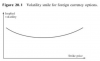# R40-P2-T5 Hull Problem 20.3 Volatility smile & Jumps in asset price

#### Karim_B

##### Active Member
Subscriber
Hi @David Harper CFA FRM
In R40-P2-T5 Hull - Problem 20.3 on volatility smile & Jumps in asset price I'm confused since when I read the part about Jumps in the asset price, it made me think of the learning objective below and a volatility frown.

However the answer to the problem is a normal (happy looking?) volatility smile.

Are they referring to 2 different kinds of jumps?
1. Vol frown = A single expected up or down jump but don't know which way it will go so at the money options are most volatile
2. Vol smile = more random, larger & frequent jumps which make the distribution tails heavier.

Problem 20.3

What volatility smile is likely to be caused by jumps in the underlying asset price? Is the pattern
likely to be more pronounced for a two-year option than for a three-month option?

Jumps tend to make both tails of the stock price distribution heavier than those of the lognormal
distribution. This creates a volatility smile similar to that in Figure 20.1. The volatility smile is
likely to be more pronounced for the three-month option.

Figure 20.1Learning objective I was thinking of:

Explain the impact of asset price jumps on volatility smiles.
This concerns a situation where a large jump—either up or down—is anticipated. In such a case, the actual distribution is not lognormal but rather bimodal; i.e., it has two camel-like humps. Under such a distribution, an at-the-money option has a higher volatility than both an out-of-the-money option and an in-the-money option.
The implication of this inverted situation is an upside-down volatility smile that peaks in the middle, where the strike price equals the stock price; this is also known as a “volatility frown.”

Thanks
Karim

#### David Harper CFA FRM

##### David Harper CFA FRM
Staff member
Subscriber
@Karim_B oh okay right see, well this weekend I'll probably revisit this and see if can make in mental progress (sometimes a fresh looks adds something). Thanks!

##### Active Member
There are at least two conditions for an asset price to have a lognormal distribution
o Constant volatility of the asset
o Smooth price change without jumps (i.e., a diffusion process)
 But neither is satisfied for an exchange rate
o Volatility of FX rate is non-constant
o Exchange rates exhibit frequent jumps

The impact of these jumps and non-constant volatility depends on the option maturity. As the maturity of the option increases, the percentage impact of non-constant volatility on prices becomes more pronounced, but its percentage impact on implied volatility usually becomes less pronounced. The percentage impact of jumps on both prices and the implied volatility becomes less pronounced as the maturity of the option is increased. The result of all this is that the volatility smile becomes less pronounced as option maturity increases.

#### David Harper CFA FRM

##### David Harper CFA FRM
Staff member
Subscriber
Hi @Jaskarn Hull says a few times in his chapter that "The shape of the volatility smile depends on the option maturity. As illustrated in Table 20.2, the smile tends to become less pronounced as the option maturity increases." What is means is that shorter maturity options tend to exhibit a "bigger" smile than longer maturity. For example, if the smile for a 3 month option is σ = 30% (@ K/S = 0.75), σ = 21% (ATM), and σ = 25% (@ K/S = 1.25); then maybe the longer-term option at one-year isn't such a big grin, maybe it is: σ = 25% (@ K/S = 0.75), σ = 21% (ATM), and σ = 22% (@ K/S = 1.25). I don't think he ever explains why this tends to be observed ... Thanks,